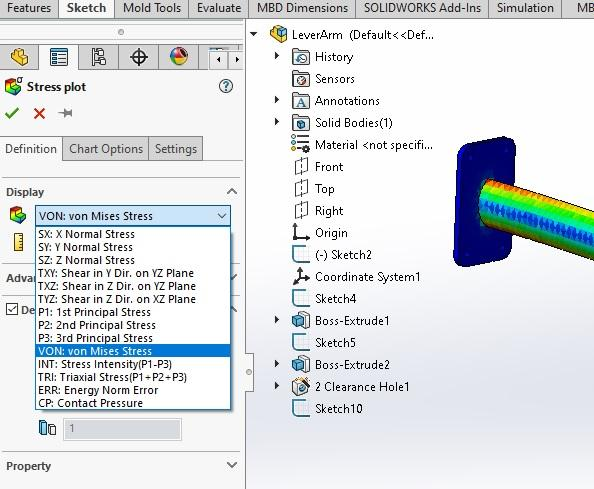# Flexure Stress: Manual vs CAD Simulation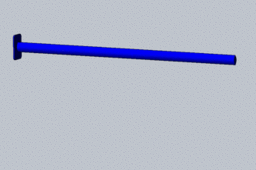Flexure Stress in a Function Defined Cross-section Cantilever
Manual Calculations vs. Simulation in SolidWorks

1. ### Step 1: Cantilever beam Defined

Given: A 10.0-meter-long beam that has a cross-section defined by the function Y^2=400x, made of ASTM A-36 steel and is to carry a 5.0 kN load on the end of the beam.

The bending stress in the beam is due to the weight of the beam plus the 5.0 kN weight on the unrestrained end as shown.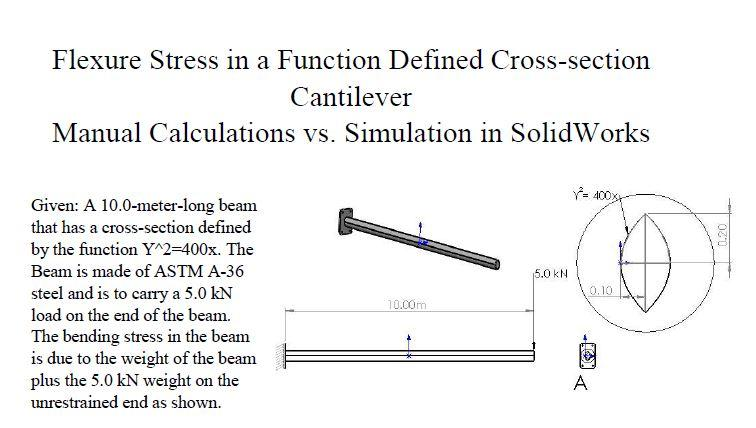2. ### Step 2: Calculate Area and y-bar

Manual Calculations:

1-     Determine the area and Y-bar using calculus of the proposed lever arm.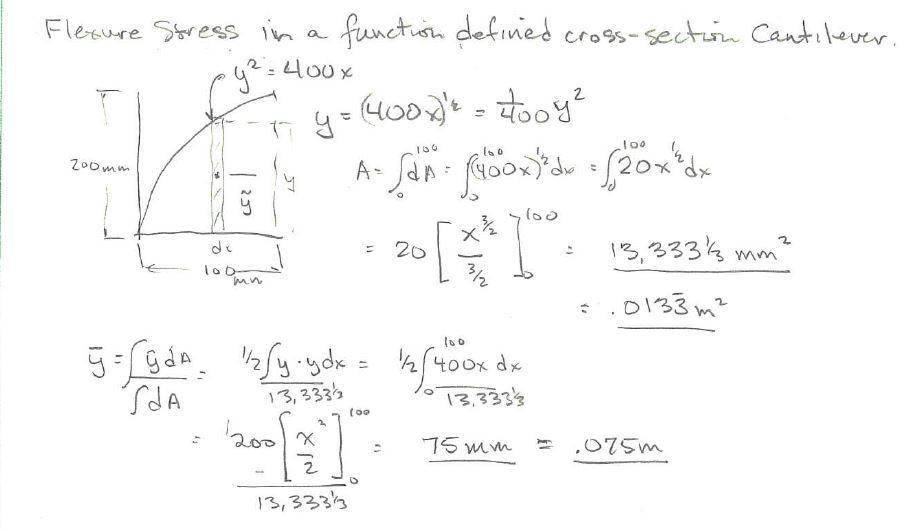Determine the Area Moment of Inertia using calculus of the proposed lever arm.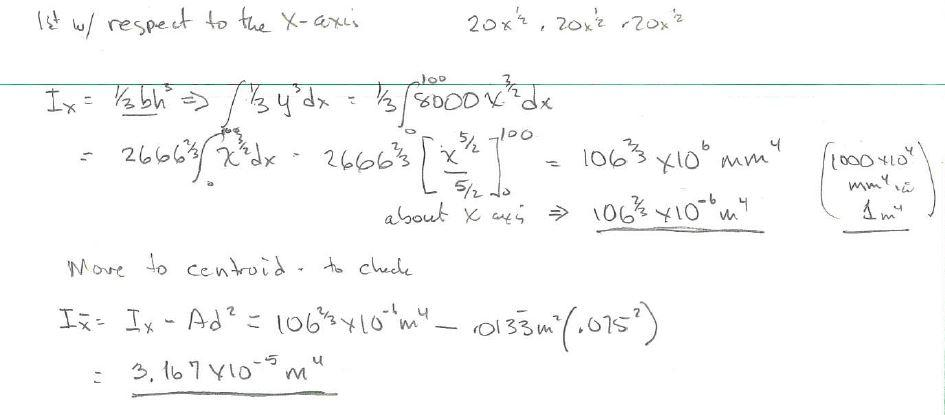The Section Modulus , S = I/C of this quarter Section (which we will not be using) is 106.7X10^-4 / .075 = .142 m^3. You would only need this is you want to use this profile as the beam cross-section.

3. ### Step 3: Calculate Area Moment of Inertia

Area Moment of Inertia is defined as the Second Moment of an Area.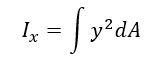In this case we want the Area Moment of Inertia with respect to the X-axis and then multiple that value by 4 for the full cross-section of the beam. The value of the Moment of Inertia with respect the the Centroidal X-axis is only given in this case as a check in SolidWorks to help validate our manual calculations since SolidWorks Section properties only calculates Area Moment of Inertia with respect to the centroidal axes.So the Area moment of Inertia and area of the complete cross-section is shown: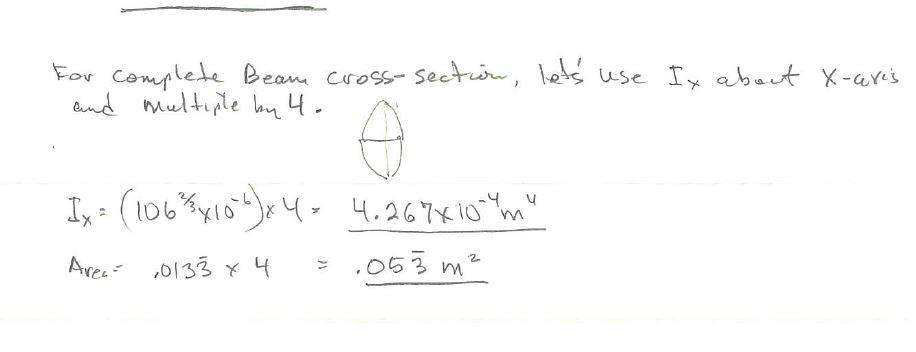4. ### Step 4: Calculate the beam weight

The volume of the beam is the area X length.

The mass = Volume X Density

The weight, W = mass X acceleration due to gravity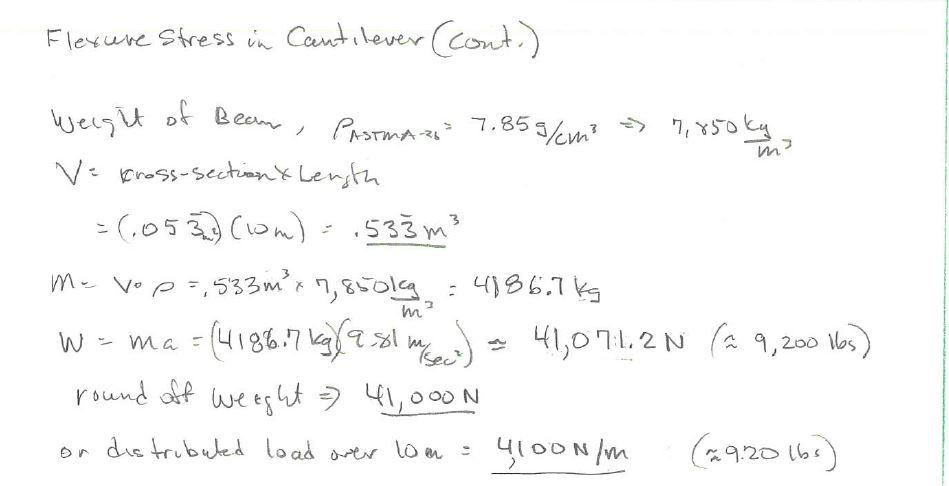5. ### Step 5: Shear and Bending Moment Diagrams

Now we can sketch the Shear and bending Moment Diagrams due to the weight of the beam and the 5.0 kN load on the end of the beam.

From this we determine the maximum moment of 255 kN-m occurs at the restraint as we can expect with a cantilever.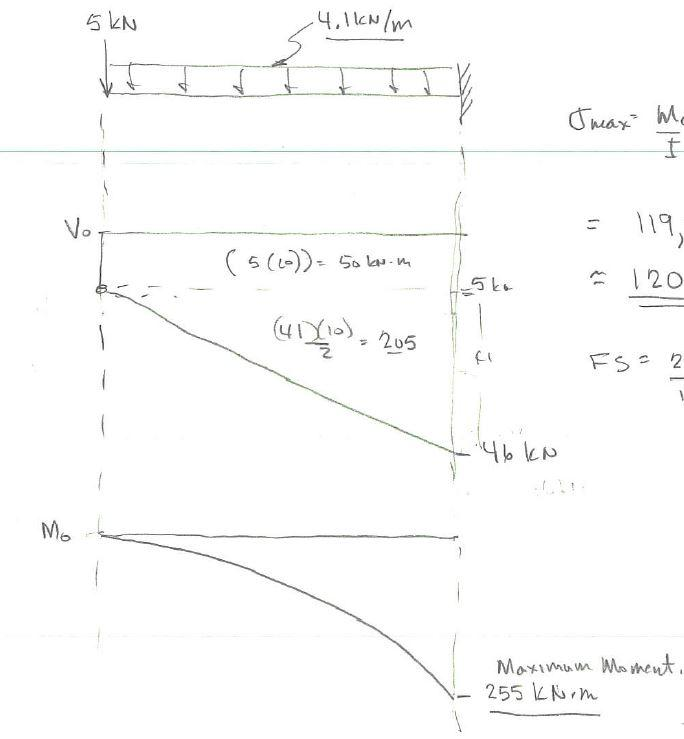6. ### Step 6: Calculate flexure stress

So the maximum bending stress is approximately 120 Mpa with a factor of safety of about 2.1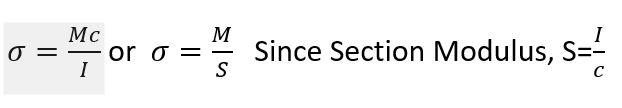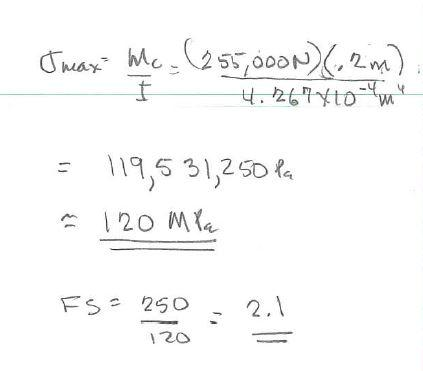7. ### Step 7: Equation Driven Curve

Now, in SolidWorks we can create the beam by first creating the function defined cross-section by inserting an Equation Driven Curve

with the parameters and syntax as shown below in the dialogue box.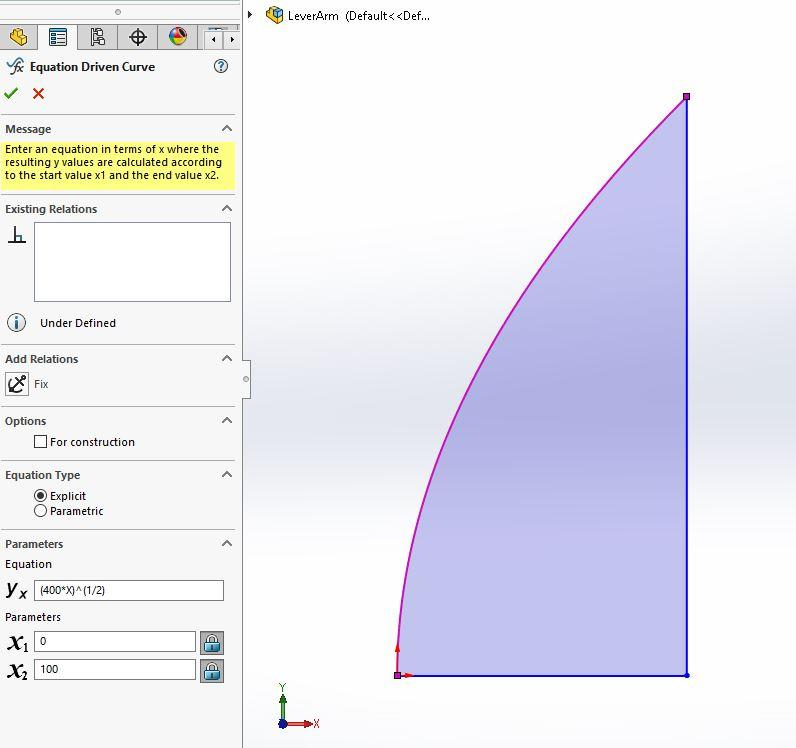8. ### Step 8: Check the section properties

Make sure the area, centroids, and Area Moment of Inertia matches the manual calculations of the one-quarter section of the beam. This is just to give you confidence as those values are calculated manually for other function defined cross-sections.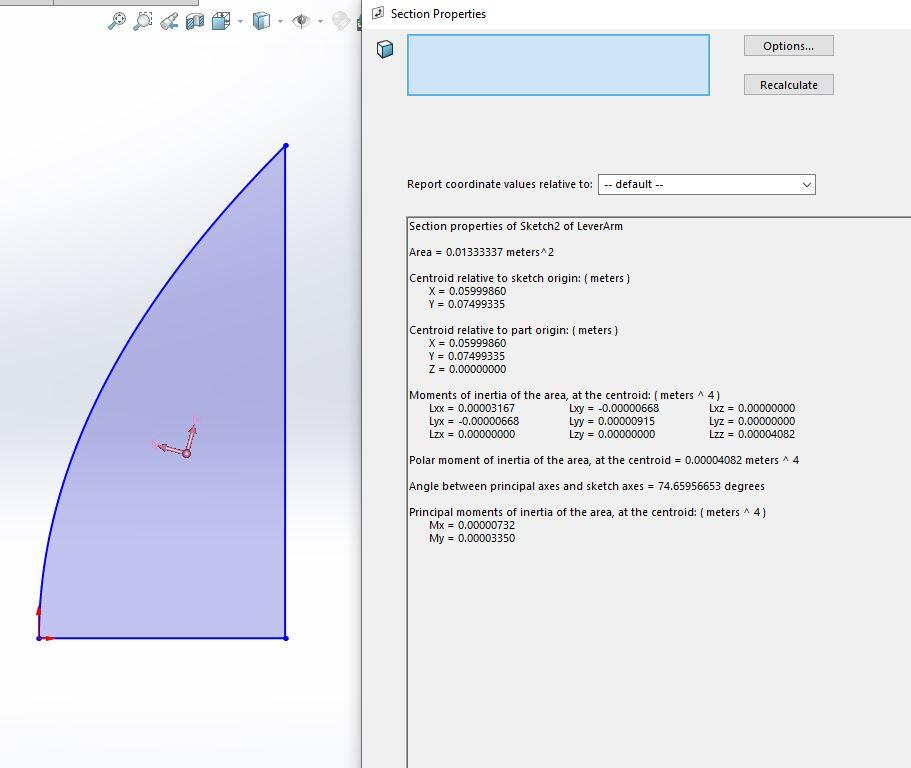9. ### Step 9: Create the 3D solid model of the beam

Mirror the quarter section and then mirror again those profiles to create the complete cross-section of the beam. Now extrude this cross-section so it is 10.0 meters long.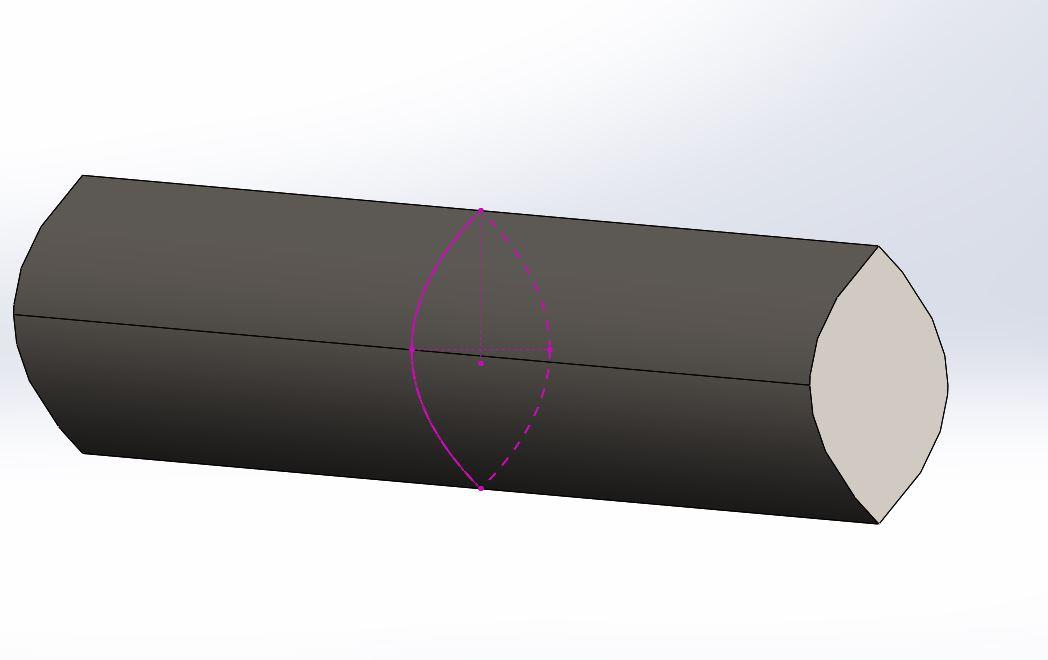10. ### Step 10: Check the mass properties of the beam

Make sure the volume and mass of the beam matches the manual calculations.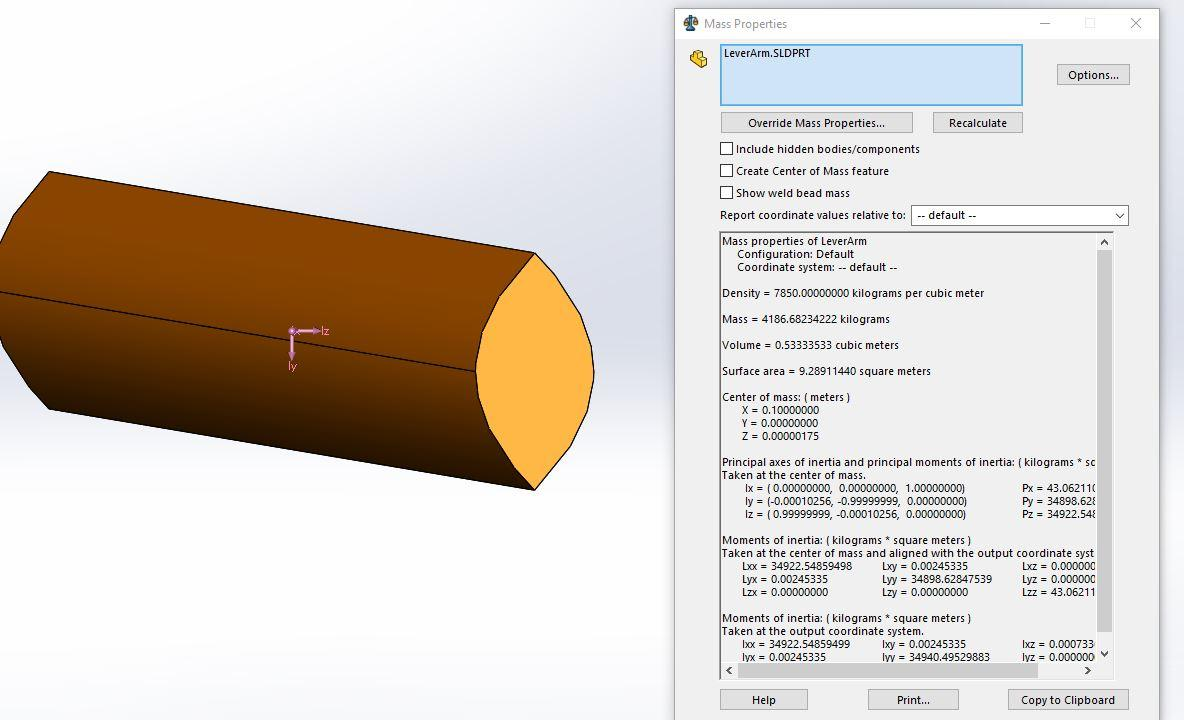11. ### Step 11: Add a plate on restrained end for visual effects

An end plate can be added to the restrained end if you would like just to help with visualization of the simulation.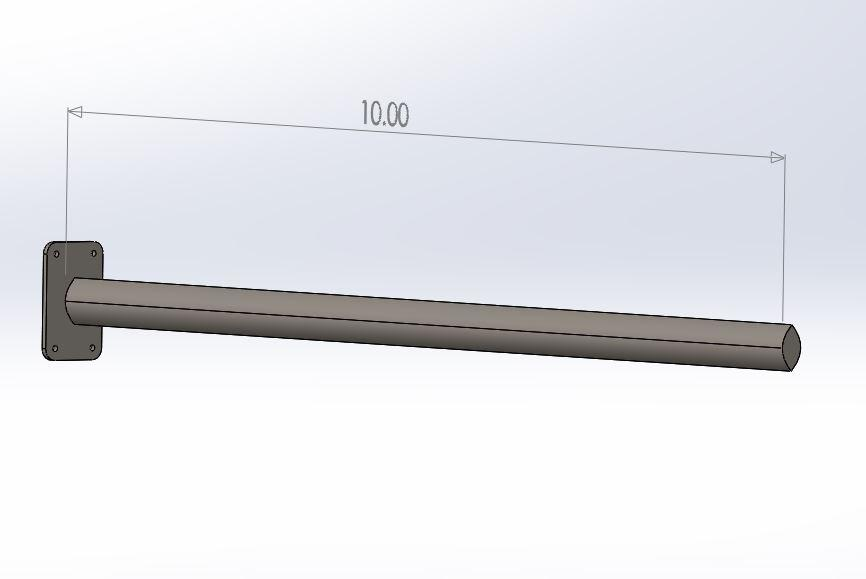12. ### Step 12: Setup Simulation

This is assuming you have the Simulation package in SolidWorks already loaded and ready to use. (See Axial Deformation Tutorial if this is not the case.)

From the Pull-down Menus, Select Simulation, Static Analysis.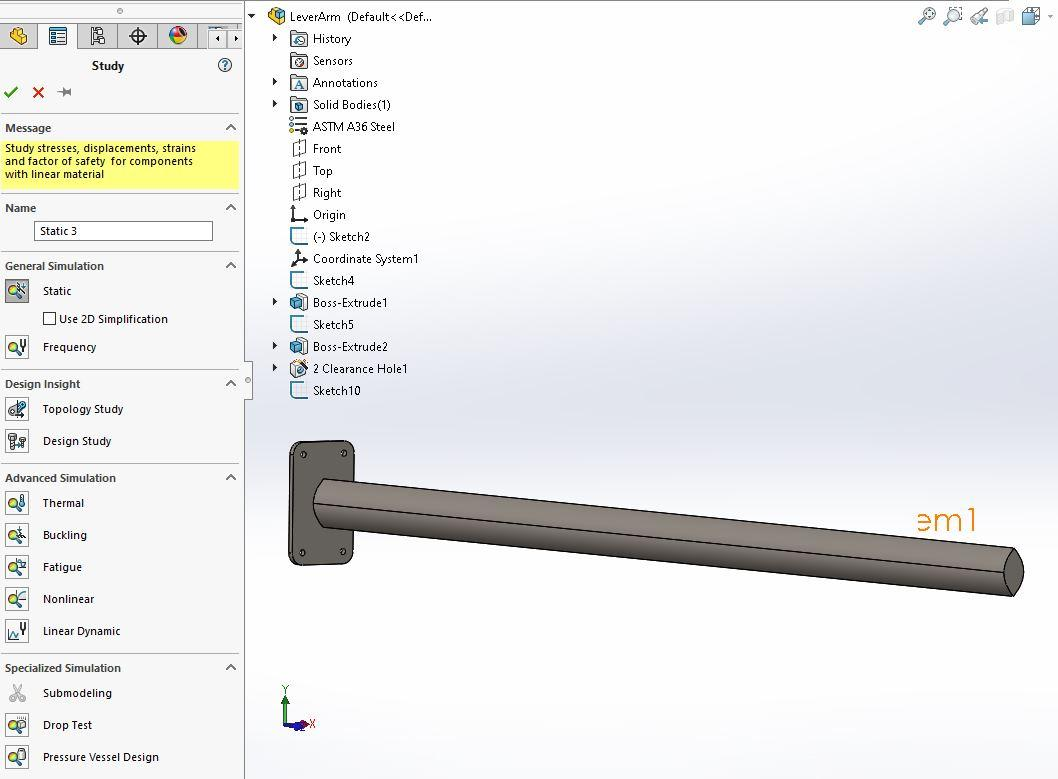From the Pull-down Menus, Select Simulation, Apply Material to all. Select ASTM A-36 material and then select Apply and Close.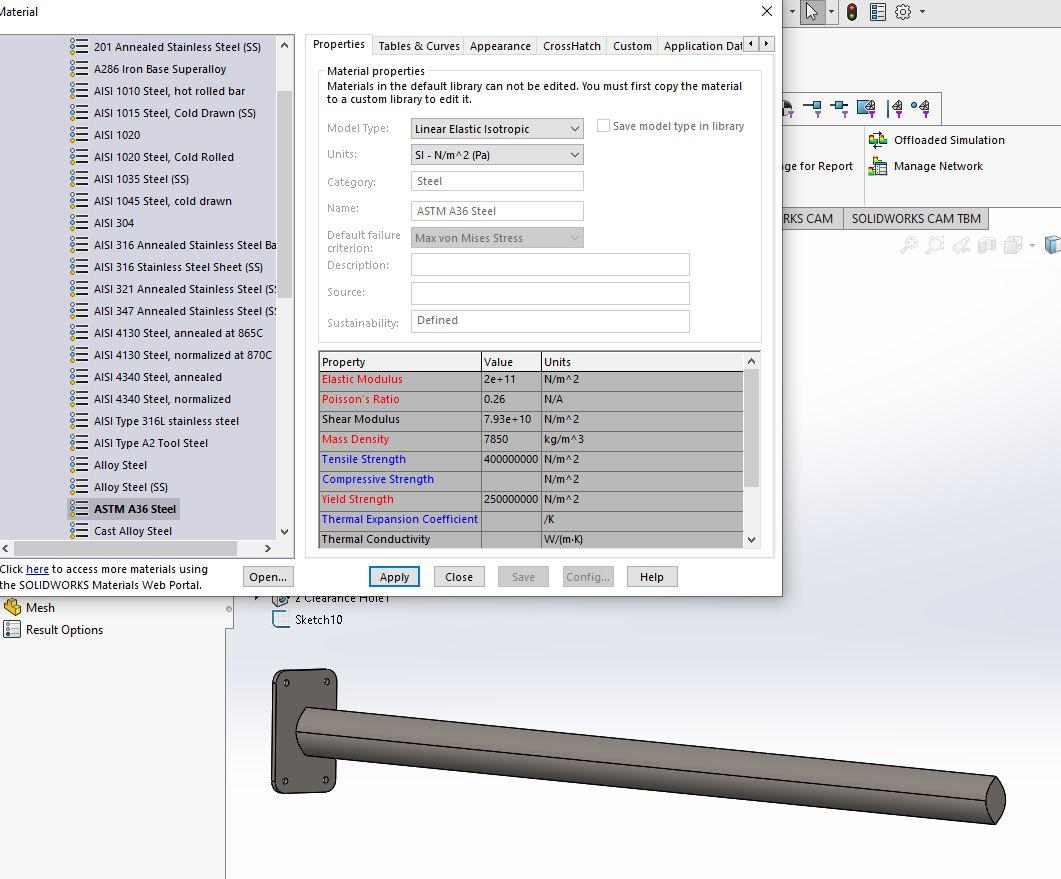From the Pull-down Menus, Select Simulation, Loads/Fixtures, Fixtures, Fixed Geometry. The Select the stationary end of the cantilever as shown below.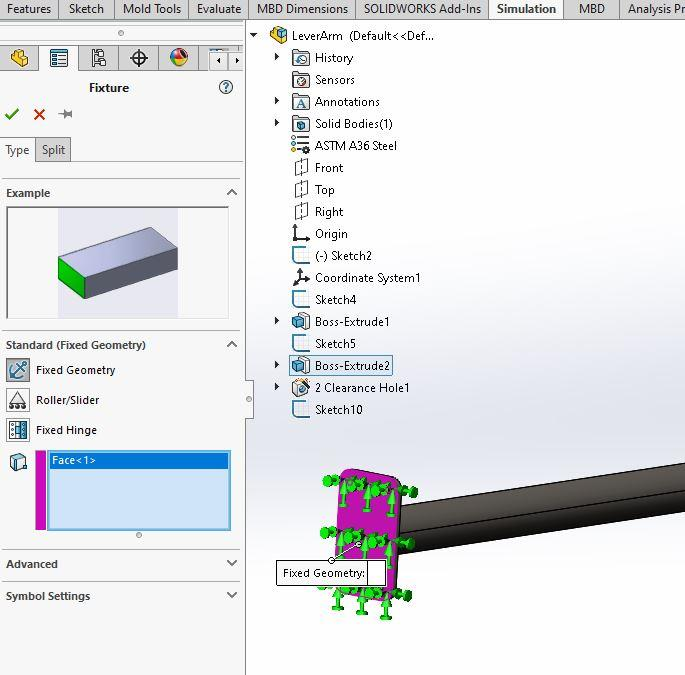From the Pull-down Menus, Select Simulation, Loads/Fixtures, Force. Then in the dialogue box, select Force and with the box for 'Faces and Edges for Normal Force' highlighted, pick the top vertex line of the Cantilever where the weight of the beam is to be placed so the top edge is now indicated in the dialogue box.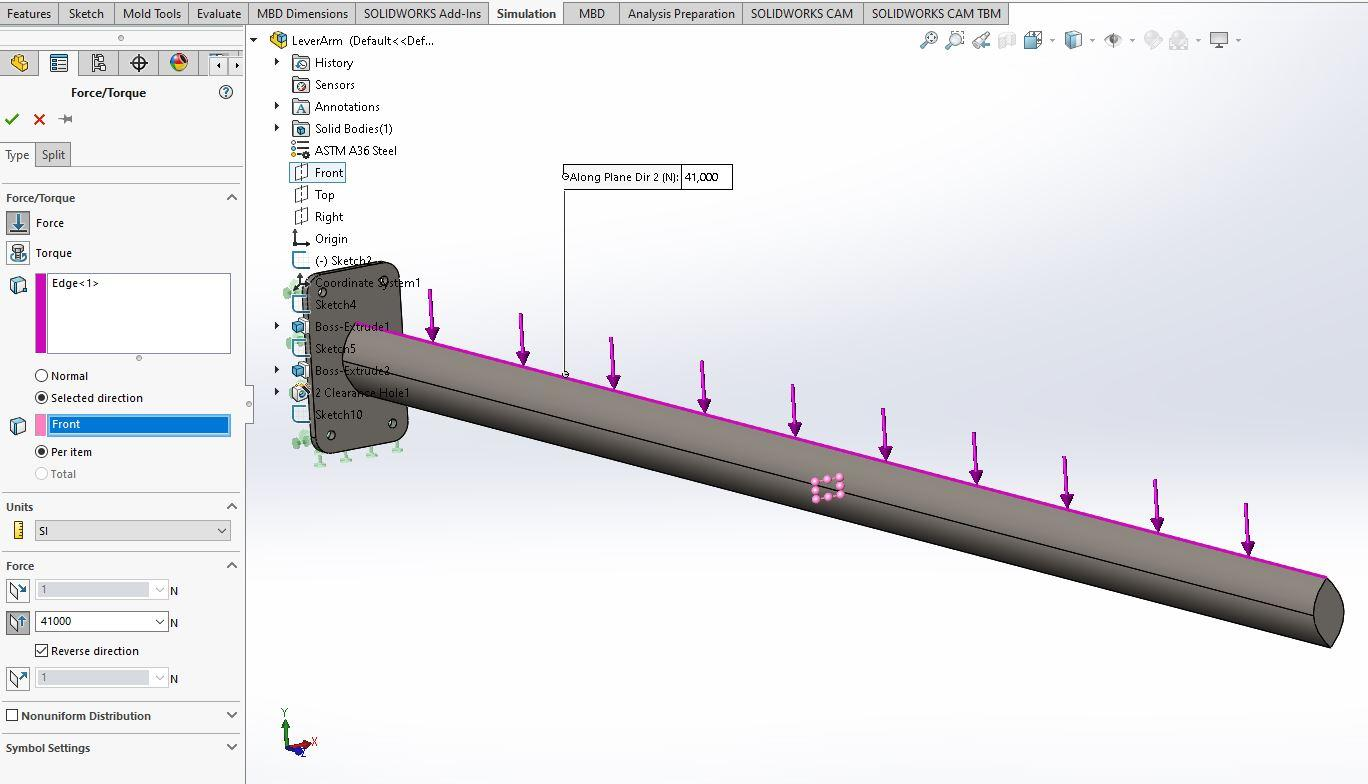Pick the Selected direction option and then pick the Front Plane from the model's Feature Manager Tree (this is assuming the profile of the extruded beam is on the Front Plane of the model which is the default plane when creating an Equation Driven Curve where Y is a function of X) the Force direction (second position in the list) for a vertical force direction and then type in the total weight of the beam ( 41,000 N) and then pick Reverse Direction to get the force in the negative Y direction.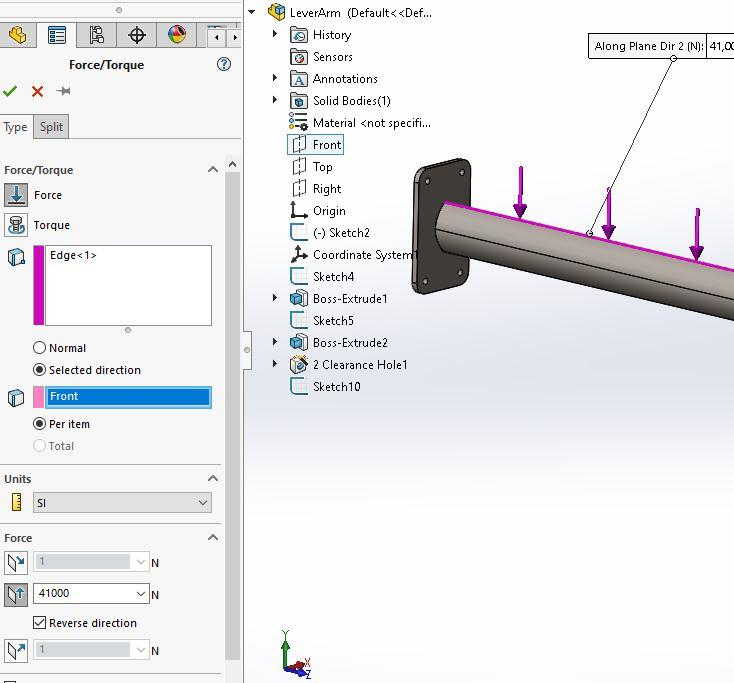Now, place the 5.0 kN load on the end of the Beam using a similar procedure as above. From the Pull-down Menus, Select Simulation, Loads/Fixtures, Force. Then in the dialogue box, select Force and with the box for 'Faces and Edges for Normal Force' highlighted, pick the end point of the Cantilever where the 5.0 kN load is be placed so the end vertex is now indicated in the dialogue box. Use the same procedure used above to get the force pointing in the negative Y direction.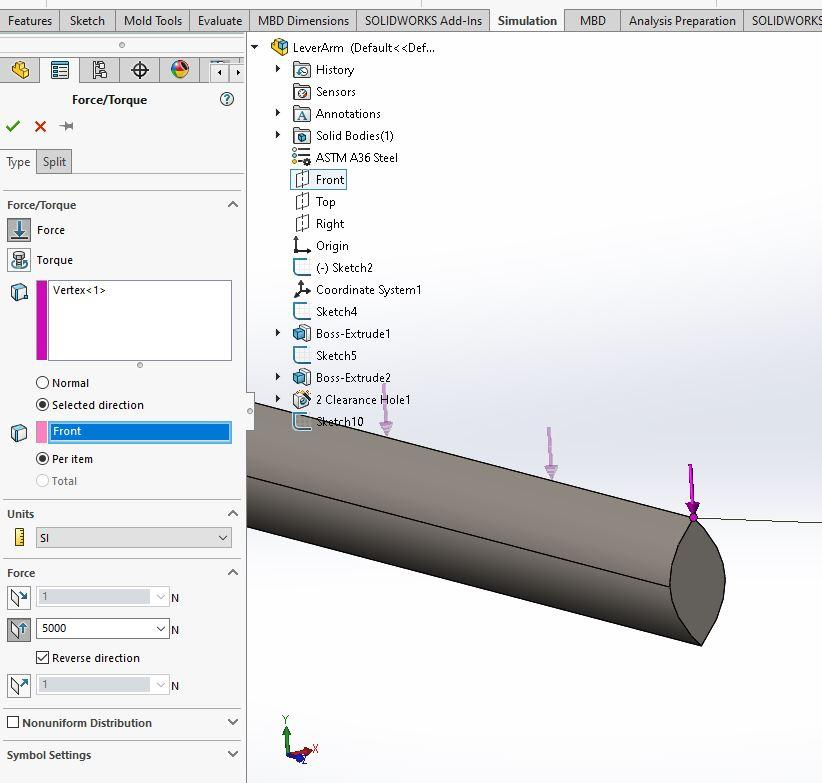14. ### Step 14: Mesh the Cantilever Solid model

From the Pull-down menus, Select Mesh, Apply Control. When the dialogue box opens, select the name of your model at the upper left corner of the graphics window so that the model name appears in the dialogue box labeled 'Selected Entities.' The Mesh Density may be increased some by sliding the density indicator slider to the right about half way between the mid point and the Fine point. Select ok.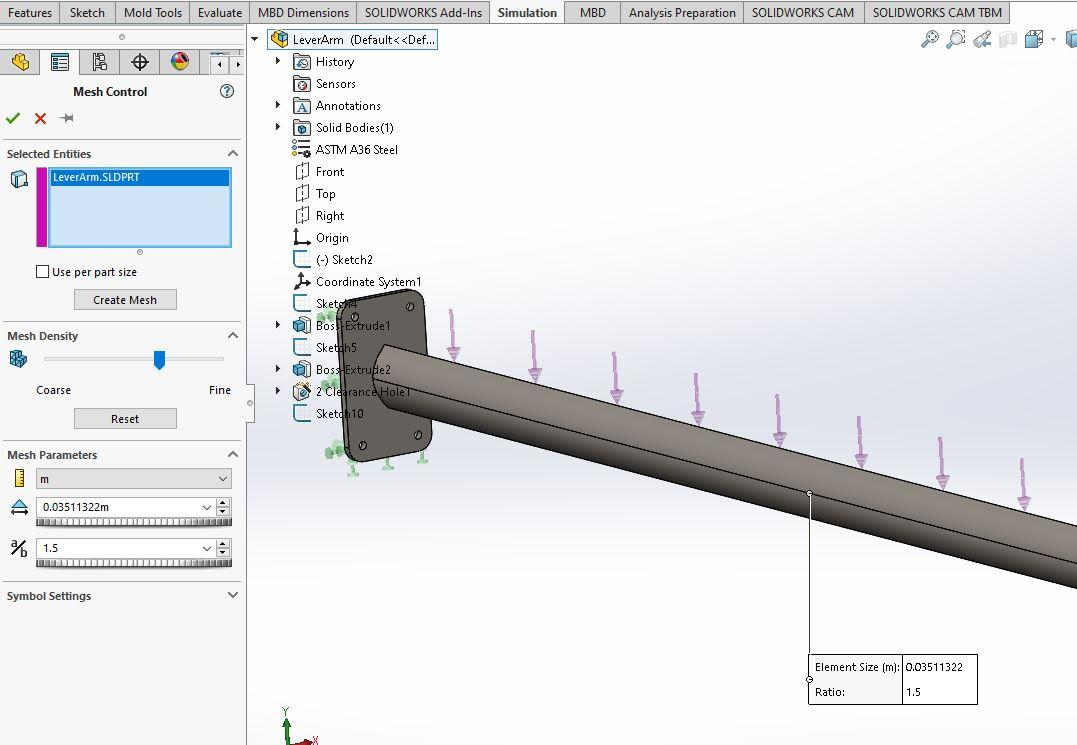Now, from the Pull-down menus, Select Mesh, Mesh Create. From the open dialogue box, the Mesh Density may again be increased by sliding the indicator slightly to the right. Select ok.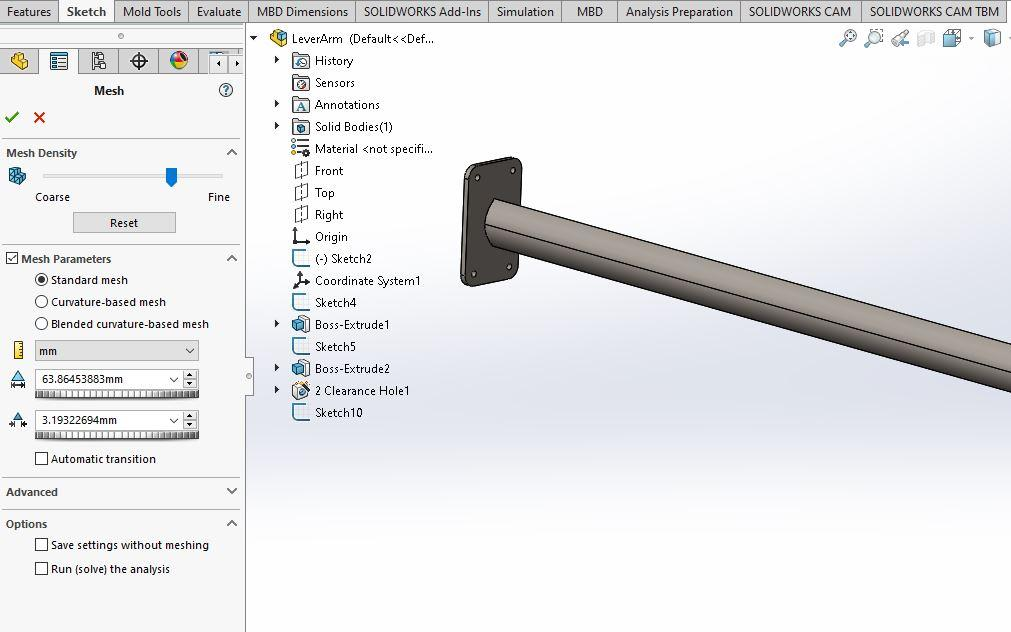The mesh is now created in the entire cantilever beam and is ready to run the simulation.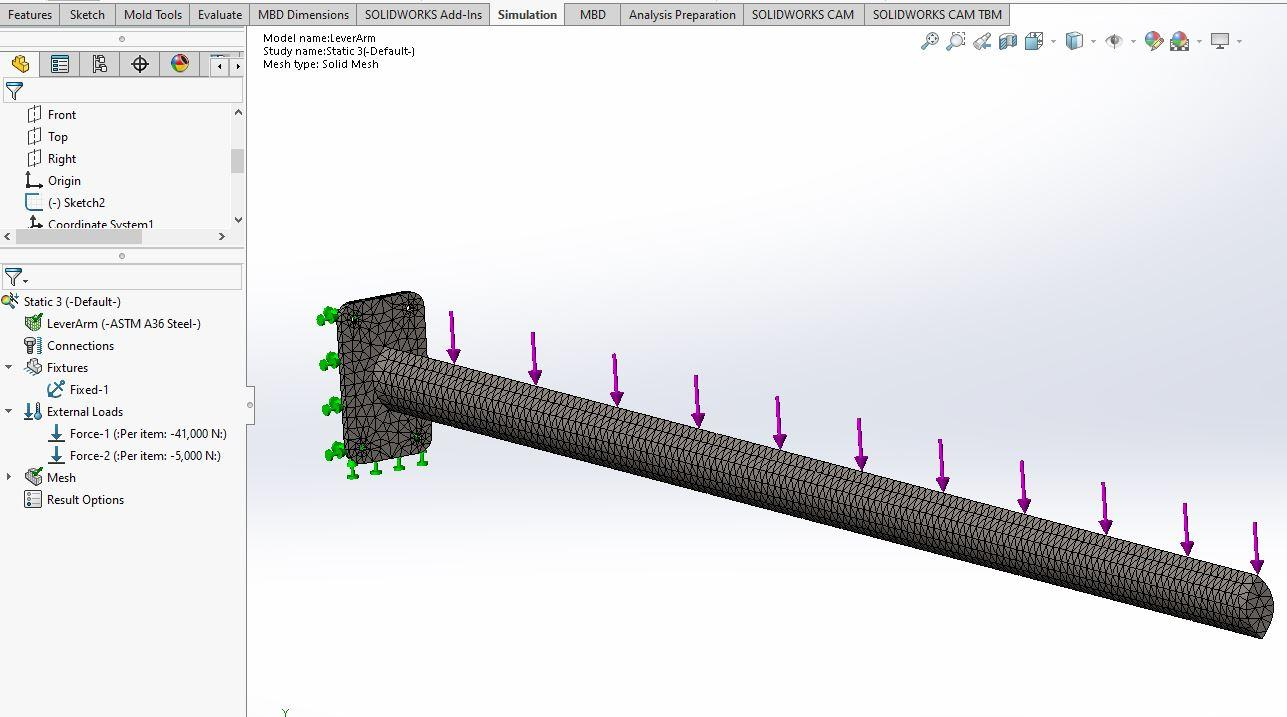15. ### Step 15: Run the Simulation

The stess is shown in the von Mises stress color plot.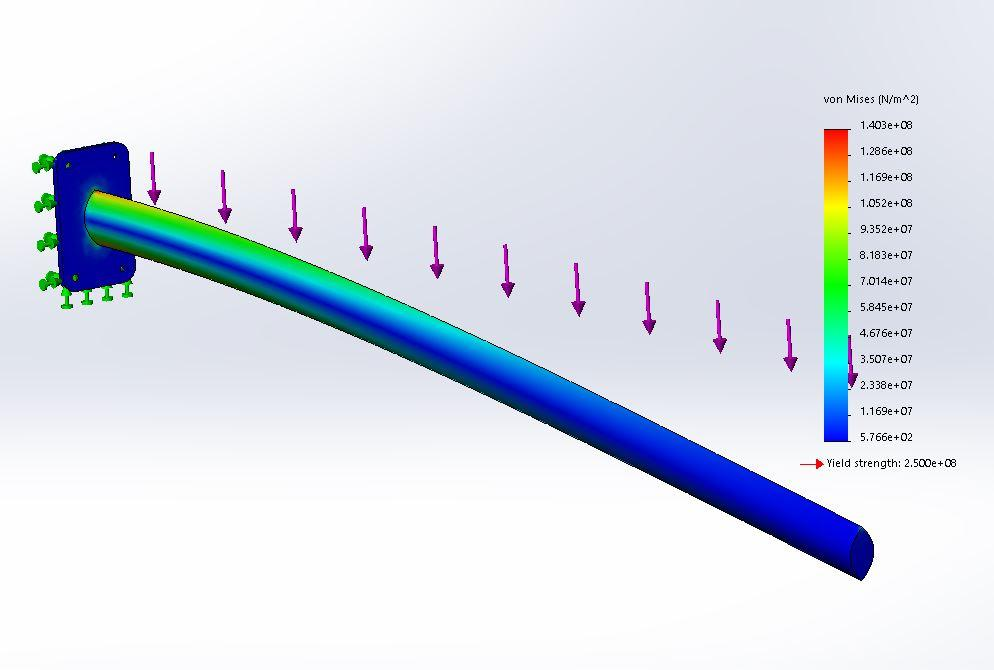Though the stress types may be changed to show the principle stresses or other stresses but since there are no other forces such as torque the combined stresses would not change significantly.